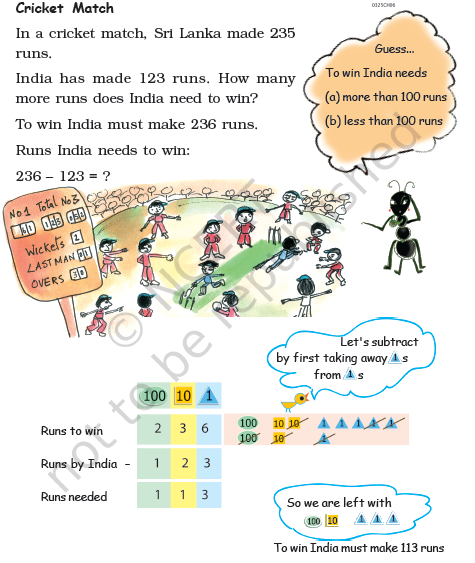# NCERT Class 3 Maths Give and Take

Read and download NCERT Class 3 Maths Give and Take chapter in NCERT book for Class 3 Mathematics. You can download latest NCERT eBooks for 2022 chapter wise in PDF format free from Studiestoday.com. This Mathematics textbook for Class 3 is designed by NCERT and is very useful for students. Please also refer to the NCERT solutions for Class 3 Mathematics to understand the answers of the exercise questions given at the end of this chapter

## Give And Take Class 3 Mathematics NCERT

Class 3 Mathematics students should refer to the following NCERT Book chapter Give And Take in standard 3. This NCERT Book for Grade 3 Mathematics will be very useful for exams and help you to score good marks

### Give And Take NCERT Class 3Please refer to attached file for NCERT Class 3 Maths Give and Take

 NCERT Class 3 Maths Where to Look From
 NCERT Class 3 Maths Play with Patterns
 NCERT Class 3 Maths Jugs and Mugs
 NCERT Class 3 Maths Can We Share
 NCERT Class 3 Maths Smart Charts
 NCERT Class 3 Maths Rupees and Paise
 NCERT Class 3 Maths Fun with Numbers
 NCERT Class 3 Maths Give and Take
 NCERT Class 3 Maths Long and Short
 NCERT Class 3 Maths Shapes and Designs
 NCERT Class 3 Maths Fun with Give and Take
 NCERT Class 3 Maths Time Goes On
 NCERT Class 3 Maths Who is Heavier
 NCERT Class 3 Maths How Many Times